## Loren on the Art of MATLABTurn ideas into MATLAB

Note

Loren on the Art of MATLAB has been archived and will not be updated.

# ODE Solver Selection in MATLAB

Today, I'd like to welcome Josh Meyer as this week's guest blogger. Josh works on the Documentation team here at MathWorks, where he writes and maintains some of the MATLAB Mathematics documentation. In this post, Josh provides a bit of advice on how to choose which ODE solver to use. Over to you, Josh...

### Contents

#### Initial Value Problems

There are 7 ordinary differential equation initial value problem solvers in MATLAB:

• ode45
• ode23
• ode113
• ode15s
• ode23s
• ode23t
• ode23tb

(note that ode15i is left out of this discussion because it solves its own class of initial value problems: fully implicit ODEs of the form $f(t,y,y') = 0$)

To choose between the solvers, it's first necessary to understand why one solver might be better than another for a given problem.

The ODE solvers in MATLAB all work on initial value problems of the form,

$$y' = f \left( t,y \right)$$

where $y' = dy/dt$. There is also a more general form,

$$M(t,y) y' = f \left( t,y \right)$$

where $M(t,y)$ is referred to as the mass matrix.

Starting with the initial conditions $y_0$, and a period of time over which the answer is to be obtained $(t_0,t_f)$, the solution is obtained iteratively by using the results of previous steps according to the solver's algorithm. At the first such step, the initial conditions provide the necessary information that allows the integration to proceed. The final result is that the ODE solver returns a vector of time steps $t_0,t_1,...,t_f$ as well as the corresponding solution at each time step $y_0,y_1,...,y_f$.

Theoretically, this numerical solution technique is possible because of the connection between differential equations and integrals provided by the fundamental theorem of calculus:

$$y(t + h) = y(t) + \int_t^{t+h} f\left( s,y(s) \right) ds$$

The problem of calculating $y(t+h)$ becomes a question of how to approximate the integral on the right hand side. This is where different solvers come in. Each different solver evaluates the integral using different numerical techniques, and each solver makes trade-offs between efficiency and accuracy.

#### Example: Euler's Method

Euler's method is a simple ODE solver, but it provides an illustration of the trade-offs between efficiency and accuracy in an ODE solver algorithm. Suppose you want to solve

$$y' = f(t,y) = 2t$$

over the time span $[0,3]$ using the initial condition $y_0 = 0$. Each step of Euler's method is computed with

$$\begin{array}{cl} y_{n+1} &= y_n + h f \left(t_n,y_n\right)\\ t_{n+1}&= t_n + h\end{array}$$

Using $h=1$, the solution requires just three steps:

$$\begin{array}{cl} y_1 &= y_0 + f\left(t_0,y_0\right)=0\\y_2 &= y_1 + f\left(t_1,y_1 \right)=2\\y_3 &= y_2 + f\left(t_2,y_2 \right)=6 \end{array}$$

... But is it accurate?

Not really. The exact solution to this equation is

$$y(t) = t^2$$

Reducing the step size $h$ can improve the accuracy of the answer a bit, but it also requires more steps to achieve the solution. To see this, the below code solves this problem using Euler's method and compares the answer to the analytic solution for several different values of $h$.

clear, clc
h = 1;
tspan = [0 3];
f = @(t,y) 2*t;
dydt(1) = 0;
t(1) = 0;

y = @(t) t.^2;
x = linspace(0,tspan(end));
figure
plot(x,y(x))
xlabel('t'), ylabel('y(t)')
hold on

while h > 0.1
for k = 2:tspan(end)/h+1
dydt(k) = dydt(k-1) + h*f(t(k-1),dydt(k-1));
t(k) = t(k-1) + h;
end

plot(t,dydt,'-o')
h = h/2;
end
legend('Exact Solution', 'h=1','h=0.5','h=0.25','h=0.125',...
'Location','NorthWest')
title('Solution of y''=2t using Euler''s method with several step sizes')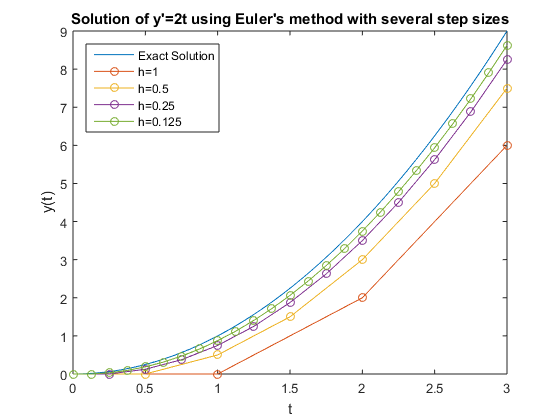#### Improving on Euler's Method

Using smaller and smaller step sizes turns out to not be a good idea, since the algorithm loses efficiency. For any reasonable problem such a solver would be very slow. Also, Euler's method has a few inherent problems. Since the slope of $y$ is evaluated only once at the beginning of each interval, this solver only produces exact answers for constant functions. There is also no way to estimate the error, so the solver needs to use fixed step sizes.

So, one way to improve on Euler's method is to evaluate $y'$ more often in each step. This provides intermediate slopes that give a better idea of what the function is doing within each interval, allowing the solver to produce exact answers for higher order problems. For example, if you add an evaluation of the slope halfway across each interval to Euler's method, then the result is called the midpoint rule, which produces exact integrations for linear functions:

$$\begin{array}{cl} s_1 &= f(t_n,y_n)\\ s_2 &= f\left( t_n + \frac{h}{2}, y_n + \frac{h}{2}s_1\right)\\y_{n+1} &= y_n + hs_2\\t_{n+1} &= t_n+h\end{array}$$

If you evaluate the slope four times in each interval, you get the classical Runge-Kutta algorithm (a.k.a. RK4), which is a piece of the ode45 algorithm. This algorithm produces exact integrations for cubic functions (and if $f$ is only a function of $t$, then $s_2=s_3$ and this is the same as Simpson's rule for quadrature):

$$\begin{array}{cl} s_1 &= f(t_n,y_n)\\s_2 &= f\left(t_n+\frac{h}{2},y_n+\frac{h}{2}s_1\right)\\s_3 &= f\left(t_n+\frac{h}{2},y_n+\frac{h}{2}s_2\right)\\ s_4 &= f\left(t_n+h,y_n+hs_3\right)\\y_{n+1} &= y_n + \frac{h}{6}\left(s_1+2s_2+2s_3+s_4\right)\\t_{n+1} &= t_n + h\end{array}$$

Runge-Kutta algorithms are all single-step solvers, since each step only depends on the result of the previous step. ode45, ode23, ode23s, ode23t, and ode23tb all employ single-step algorithms. Multi-step algorithms, such as those employed by ode113 and ode15s, use the results of several past steps.

Sophisticated ODE solvers, like the ones in MATLAB, also estimate the error in each step to determine how big the next step size should be. This is another improvement over the fixed step sizes used above, since a solver that does more work per step is able to compensate by taking steps of varying size. The error estimate used to determine the step size is typically obtained by comparing the results of two different methods. MATLAB's ODE solvers follow a naming convention that reveals information about which methods they use. ode45 compares the results of a 4th-order Runge-Kutta method and a 5th-order Runge-Kutta method to determine the error. Similarly, ode23 uses a 2nd-order and 3rd-order Runge-Kutta comparison. So, in general, the smaller the number odeNN, the looser the solver's error tolerance is.

It should be no surprise, then, that ode45 obtains a very accurate answer for the equation we solved before with Euler's method. ode45 is MATLAB's general purpose ODE solver, and it is the first solver you should use for most problems.

y = @(t) t.^2;
x = linspace(0,3);
figure
plot(x,y(x))
xlabel('t'), ylabel('y(t)')
hold on
[t,y] = ode45(@(t,y) 2*t, [0 3], 0);
plot(t,y,'o')
xlabel('t'), ylabel('y(t)')
title('Solution of y''=2t using ode45')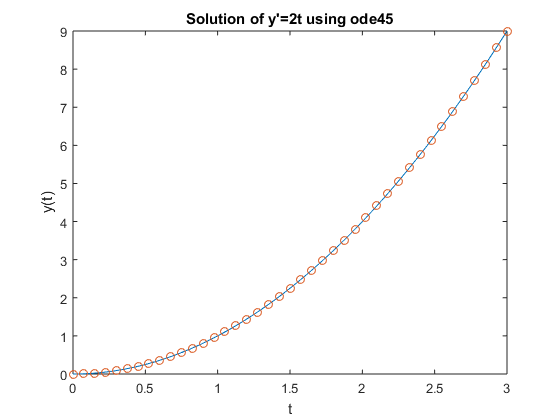#### Stiff Differential Equations

For some ODE problems, the step size taken by the solver is forced down to an unreasonably small level in comparison to the interval of integration, even in a region where the solution curve is smooth. These step sizes can be so small that traversing a short time interval might require millions of evaluations. This can lead to the solver failing the integration, but even if it succeeds it will take a very long time to do so.

Equations that cause this behavior in ODE solvers are said to be stiff. This is a nod to the fact that the equations are stubborn and not easily evaluated with numerical techniques. The problem that stiff ODEs pose is that explicit solvers (such as ode45) are untenably slow in achieving a solution. This is why ode45 is classified as a nonstiff solver along with ode23 and ode113. These solvers all struggle to integrate stiff equations.

Equation stiffness resists a precise definition, because there are several factors that cause it. Stiffness results from a combination of the specific equations, the ODE solver being used, the initial conditions, and the error tolerance used by the solver. The following statements about stiffness, attributed to Lambert , are exhibited by many examples of stiff ODEs, but counterexamples also exist, so they are not true definitions of stiffness:

1. A linear constant coefficient system is stiff if all of its eigenvalues have negative real part and the stiffness ratio [of the largest and smallest eigenvalues] is large.
2. Stiffness occurs when the mathematical problem is stable, and yet stability requirements, rather than those of accuracy, severely constrain the step length.
3. Stiffness occurs when some components of the solution decay much more rapidly than others.

A common theme among these statements is that stiffness can result from a difference in scaling somewhere in the problem. This difference in scale (for example, if the Jacobian $J = \partial f_n/\partial y_i$ has a large ratio of negative eigenvalues) constrains the step size that the solver can take in performing the integration. Tiny step sizes become necessary in order to preserve any notion of error tolerance or stability in the solution.

For example, equations describing chemical reactions frequently display stiffness, since it is common for components of the solution to vary on drastically different time scales (reactions occurring at the same time that are both very slow and very fast).

However, there are solvers specifically designed to work on stiff ODEs. Solvers that are designed for stiff problems typically do more work per step, and the pay-off is that they are able to take much larger steps and enjoy improved numerical stability compared to the nonstiff solvers. Stiff solvers are implicit, because the computation of $y$ requires the use of linear algebra to solve systems of linear equations. The Jacobian is used to estimate the local behavior of the ODE as the integration proceeds, so supplying the analytical Jacobian can improve the performance of MATLAB's stiff ODE solvers.

This is just a cursory treatment of stiffness, because it is a complex topic. See Ordinary Differential Equations: Stiffness for a more in-depth look.

To summarize, the nonstiff solvers in MATLAB are:

• ode45
• ode23
• ode113

The stiff solvers are (when ode45 is slow):

• ode15s
• ode23s
• ode23t
• ode23tb

It should be noted that nonstiff solvers do work on stiff problems, it is just that they are exceptionally slow. Similarly, solvers designed for stiff problems can work on nonstiff problems, but since they do more work per step they are less efficient than their nonstiff counterparts when that extra work isn't necessary. So equation stiffness is a matter of solver efficiency, and the goal is to strike the right balance between accuracy of the solution and work done in each step by the solver.

#### Solver Recommendations

The following recommendations are adapted from the MATLAB Mathematics documentation :

• ode45 is MATLAB's general purpose single-step ODE solver. This should be the first solver you use for most problems.

For nonstiff problems:

• ode23 is another single-step solver that can be more efficient than ode45 if the problem permits a crude error tolerance. This looser error tolerance can also accommodate some mildly stiff problems.
• ode113 is a multi-step solver, and is preferred over ode45 if the function is expensive to evaluate, or for smooth problems where high precision is required. For example, ode113 excels with orbital dynamics and celestial mechanics problems.

For stiff problems (where ode45 is slow):

• ode15s is a multi-step solver that is MATLAB's general purpose solver for stiff problems. Use ode15s if ode45 fails or struggles to complete the integration in a reasonable amount of time. ode15s is also the primary solver for DAEs, which are identified as ODEs with a singular mass matrix.
• For stiff problems with crude error tolerances, ode23s, ode23t, and ode23tb provide more efficient alternatives to ode15s since they are single-step solvers. The efficiency of ode23s can be significantly improved by providing the Jacobian, since ode23s evaluates the Jacobian in each step.
• ode23s only works on ODEs with a mass matrix if the mass matrix is constant (not time- or state-dependent).
• ode15s and ode23t are the only solvers that solve DAEs of index 1.

Here is a graphic that captures the basic recommendations. In most cases, the only choice in solver you will need to make is to use ode15s instead of ode45.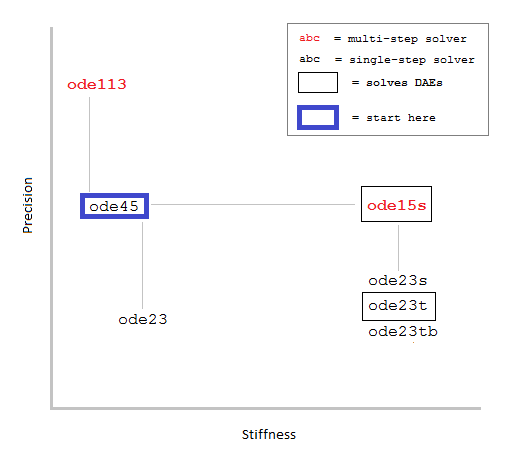#### Example 1: Damped Pendulum

The equation of motion for a damped pendulum is,

$$\ddot{\theta} = -\frac{b}{m}\dot{\theta}-\frac{mg}{L\left(m-2b\right)} \sin \theta$$

where $g$ is the gravitational constant, $m$ the mass of the bob, $L$ the length of the string, and $b$ is a damping coefficient. The goal is to solve for $\theta$, the angle that the pendulum deviates from the vertical, and $\theta '$, the rate at which the angle changes.

Some natural initial conditions would be $\theta_0 = \pi/4$ and $\theta '_0 = 0$, indicating that you lift the pendulum up to a 45 degree angle before letting go, and it has no initial angular velocity. Due to the damping coefficient, you would expect the pendulum to slowly lose momentum and go back down to rest.

The file pendulumODE.m reformulates the problem as a coupled system of first-order ODEs:

$$\begin{array}{cl} y_1' &= y_2\\y_2' &= -\frac{b}{m} y_2 -\frac{mg}{L(m-2b)}sin(y_1)\end{array}$$

then solves using ode45, ode15s, ode23, and ode113. The solutions for $y_1 = \theta$ are plotted, and the file returns the stats for each solver. As is always the case when displaying execution times, "the timings displayed can vary".

function pendulumODE
opts = odeset('stats','on');
tspan = [0 25];
y0 = [pi/4, 0];

disp(' '), disp('Stats for ode45:')
tic, [t1,y1] = ode45(@pendode, tspan, y0, opts);
toc

disp(' '), disp('Stats for ode15s:')
tic, [t2,y2] = ode15s(@pendode, tspan, y0, opts);
toc

disp(' '), disp('Stats for ode23:')
tic, [t3,y3] = ode23(@pendode, tspan, y0, opts);
toc

disp(' '), disp('Stats for ode113:')
tic, [t4,y4] = ode113(@pendode, tspan, y0, opts);
toc

figure
subplot(2,2,1), plot(t1,y1(:,1),'-o'), xlim([0 25]), title('ode45')
subplot(2,2,2), plot(t2,y2(:,1),'-o'), xlim([0 25]), title('ode15s')
subplot(2,2,3), plot(t3,y3(:,1),'-o'), xlim([0 25]), title('ode23')
subplot(2,2,4), plot(t4,y4(:,1),'-o'), xlim([0 25]), title('ode113')

function dy2dt2 = pendode(t,y)
g = 9.8; %m/s^2
m = 1;   % Mass of bob
L = 2;   % Length of pendulum in meters
b = 0.2; % Damping coefficient
dy2dt2 = [y(2); -b/m*y(2)-g/L*sin(y(1))];
end
end


pendulumODE


Stats for ode45:
75 successful steps
0 failed attempts
451 function evaluations
Elapsed time is 0.011970 seconds.

Stats for ode15s:
183 successful steps
9 failed attempts
315 function evaluations
1 partial derivatives
31 LU decompositions
311 solutions of linear systems
Elapsed time is 0.081467 seconds.

Stats for ode23:
263 successful steps
36 failed attempts
898 function evaluations
Elapsed time is 0.015948 seconds.

Stats for ode113:
159 successful steps
3 failed attempts
322 function evaluations
Elapsed time is 0.018056 seconds.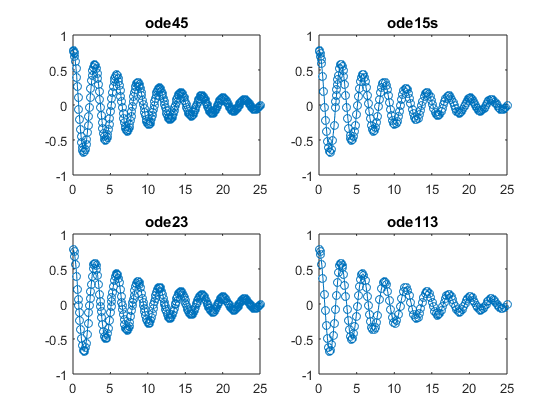The solvers all perform well, but the damped pendulum is a good example of a nonstiff problem where ode45 performs nicely. In this case ode15s needs to do extra work in order to achieve an inferior solution.

#### Example 2: van der Pol Oscillator

The van der Pol Oscillator equation becomes stiff in certain intervals when the nonlinear parameter $\mu$ is large:

$$\ddot{y} - \mu \left(1-y^2\right)\dot{y}+y=0$$

The nonlinearity of this equation is contained entirely in the term that involves $\mu$: notice that if $\mu=0$, the equation reduces to that of a simple harmonic oscillator, which has regular periodic behavior.

Attempting to solve this equation using ode45 is met with severe resistance, requiring millions of evaluations and 30+ minutes of execution (I stopped execution after 35 minutes). Since the problem is clearly stiff, this example compares the stiff solvers.

The file vanderpolODE.m finds the solution for $\mu=1000$ using ode15s, ode23s, ode23t, and ode23tb. The function file vdp1000.m ships with MATLAB and encodes this equation as a coupled system of first-order ODEs:

$$\begin{array}{cl}y_1' &= y_2\\y_2' &= \mu \left( 1-y_1^2 \right)y_2 - y_1\end{array}$$

The Jacobian is supplied to assist the solvers, and its use is reflected in the number of partial derivative evaluations.

function vanderpolODE
opts = odeset('stats','on','Jacobian',@J);
tspan = [0 3000];
y0 = [2 0];

disp(' '), disp('Stats for ode15s:')
tic, [t1,y1] = ode15s(@vdp1000, tspan, y0, opts);
toc

disp(' '), disp('Stats for ode23s:')
tic, [t2,y2] = ode23s(@vdp1000, tspan, y0, opts);
toc

disp(' '), disp('Stats for ode23t:')
tic, [t3,y3] = ode23t(@vdp1000, tspan, y0, opts);
toc

disp(' '), disp('Stats for ode23tb:')
tic, [t4,y4] = ode23tb(@vdp1000, tspan, y0, opts);
toc

figure
subplot(2,2,1), plot(t1,y1(:,1),'-o'), ylim([-4 4]), title('ode15s')
subplot(2,2,2), plot(t2,y2(:,1),'-o'), title('ode23s')
subplot(2,2,3), plot(t3,y3(:,1),'-o'), title('ode23t')
subplot(2,2,4), plot(t4,y4(:,1),'-o'), title('ode23tb')

function dfdy = J(t,y)
MU = 1000; % Nonlinear coefficient
dfdy = [         0                  1
-2*MU*y(1)*y(2)-1    MU*(1-y(1)^2) ];
end
end


vanderpolODE


Stats for ode15s:
591 successful steps
225 failed attempts
1749 function evaluations
45 partial derivatives
289 LU decompositions
1747 solutions of linear systems
Elapsed time is 0.192955 seconds.

Stats for ode23s:
741 successful steps
13 failed attempts
2251 function evaluations
741 partial derivatives
754 LU decompositions
2262 solutions of linear systems
Elapsed time is 0.092824 seconds.

Stats for ode23t:
776 successful steps
94 failed attempts
2014 function evaluations
36 partial derivatives
294 LU decompositions
2012 solutions of linear systems
Elapsed time is 0.218996 seconds.

Stats for ode23tb:
573 successful steps
93 failed attempts
2816 function evaluations
44 partial derivatives
269 LU decompositions
3415 solutions of linear systems
Elapsed time is 0.202237 seconds.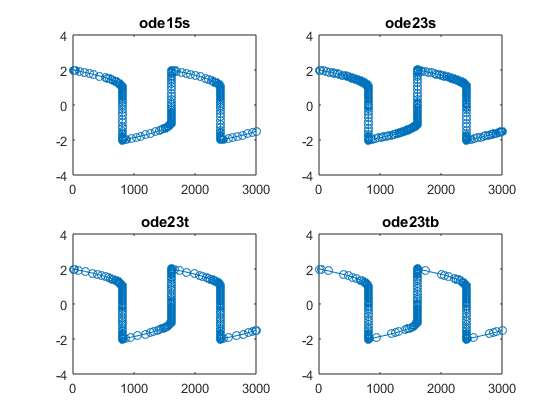The plots are of the solutions for $y_1$. For this problem, ode23s executes quickest and with the least number of failed steps. The supplied Jacobian greatly assists ode23s in evaluating the partial derivatives in each step. ode23tb also solves the problem with the fewest number of steps, outperforming ode15s. This problem is a good example of a stiff problem with a crude tolerance where ode23s and ode23tb can out perform ode15s. But practically speaking, all of the stiff solvers perform well on this problem and offer significant time savings when compared to ode45.

#### Summary

Although all of the ODE solvers are capable of working on the same problems, it's recommended that you start with ode45. Then, if the problem exhibits signs of stiffness, ode15s is a good second choice. The other solvers then offer further refinement based on the properties of the specific problem and whether extra information (such as the Jacobian) can be provided.

Does your work involve the use of MATLAB's ODE solvers? If so, share your experience here.

 C. Moler, Ordinary Differential Equations Numerical Computing with MATLAB Electronic edition: The MathWorks, Inc., Natick, MA, 2004

 Shampine, L. F. and M. W. Reichelt, The MATLAB ODE Suite SIAM Journal on Scientific Computing, Vol. 18, 1997.

 C. Moler, Ordinary Differential Equations: Stiffness Cleve’s Corner: Cleve Moler on Mathematics and Computing, 2014

 L. F. Shampine, Numerical Solution of Ordinary Differential Equations, Chapman & Hall, 1994

 Shampine, L. F., Gladwell, I. and S. Thompson, Solving ODEs with MATLAB, Cambridge University Press, 2003

 J. D. Lambert, Numerical Methods for Ordinary Differential Systems, New York: Wiley, 1992